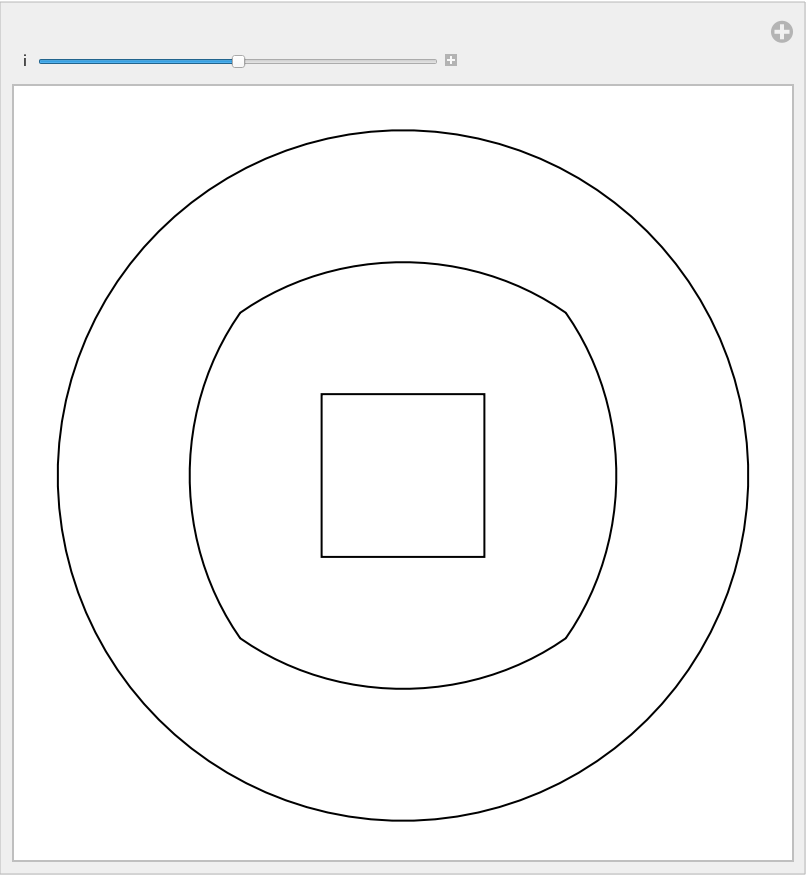#Function Repository Resource:

# BlendLine

Blend between lines

Contributed by: Sander Huisman
 ResourceFunction["BlendLine"][{line1,line2}] gives the line halfway between line1 and line2. ResourceFunction["BlendLine"][{line1,line2},α] gives the line a fraction α between line1 and line2. ResourceFunction["BlendLine"][{line1,line2,…},α] gives the line a fraction α between lines linei. ResourceFunction["BlendLine"][{line1,line2,…},{α1,α2,…}] gives several lines with fractions αi.

## Details and Options

Line objects line1 and line2 should contain a single line.
Line objects line1 and line2 can be in any dimension.

## Examples

### Basic Examples

Interpolate halfway between two lines:

 In:=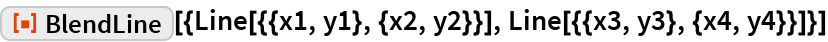Out=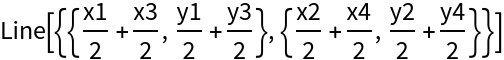Interpolate a fraction of the way between two lines:

 In:=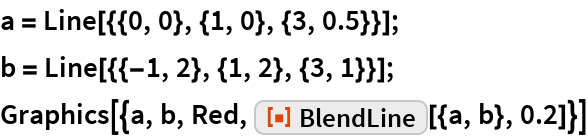Out=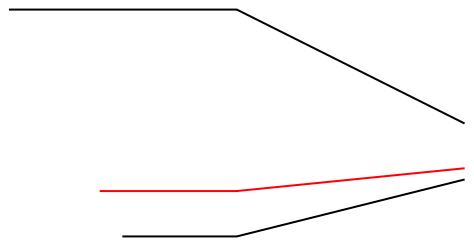### Scope

Interpolate two lines at multiple fractions:

 In:=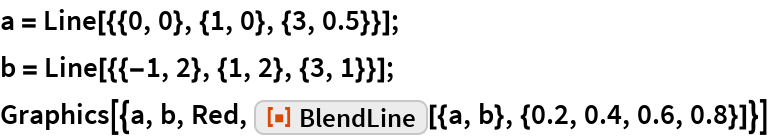Out=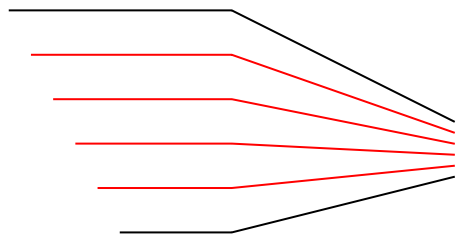Blend between three lines:

 In:=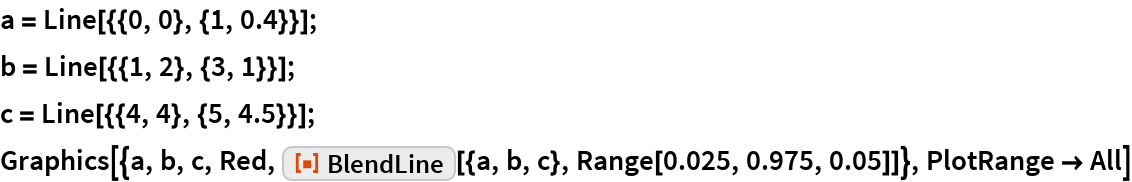Out=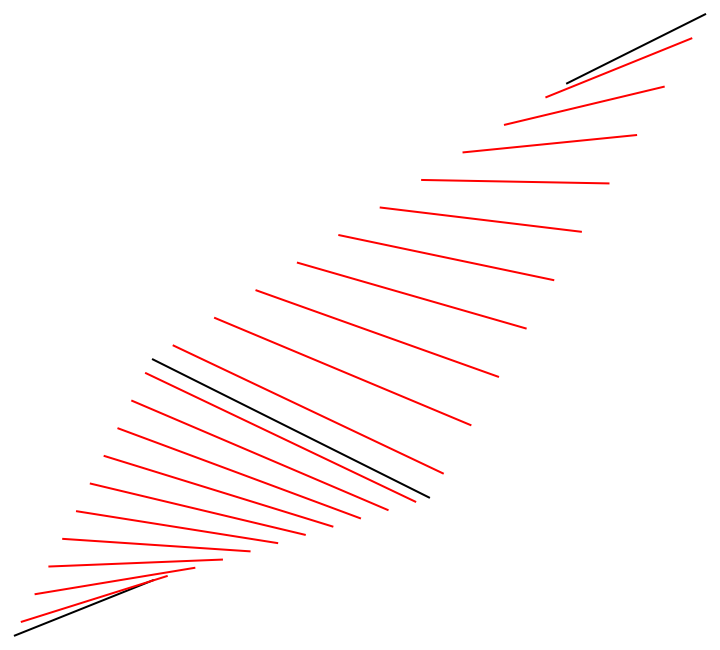Interpolate between two lines in 3D:

 In:=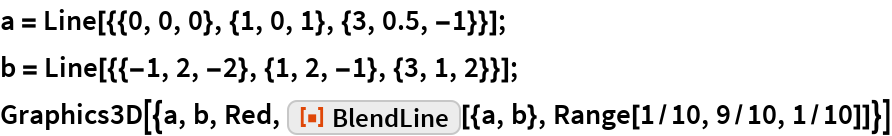Out=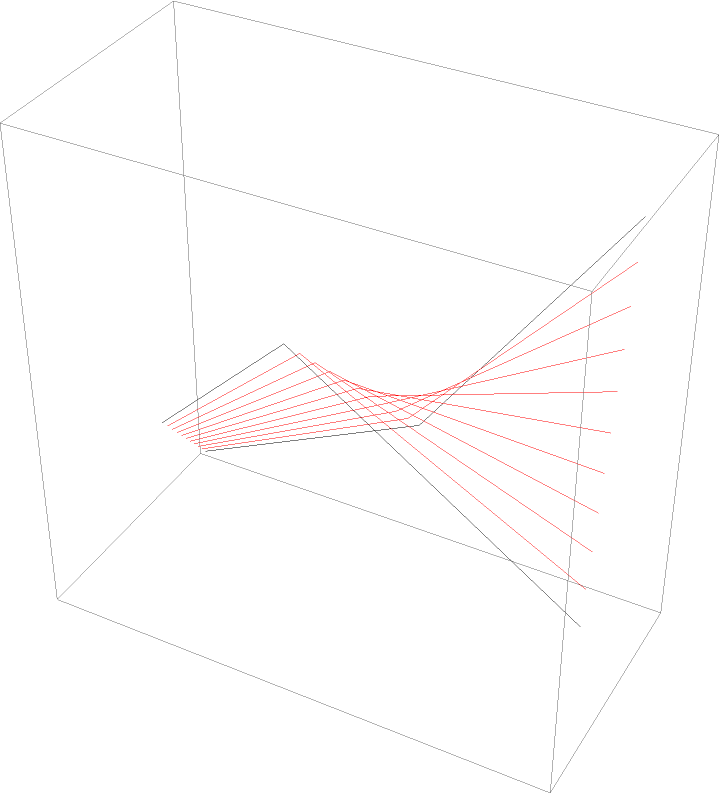Interpolate a line in 4D:

 In:=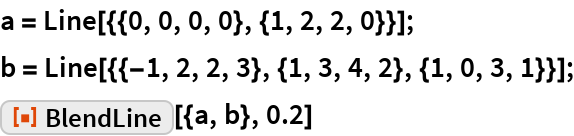Out=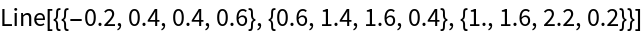### Applications

Create a grid:

 In:=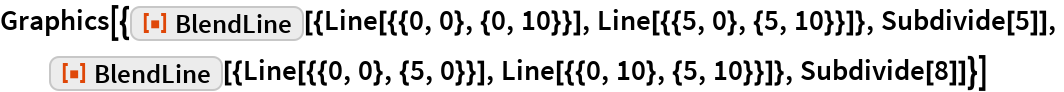Out=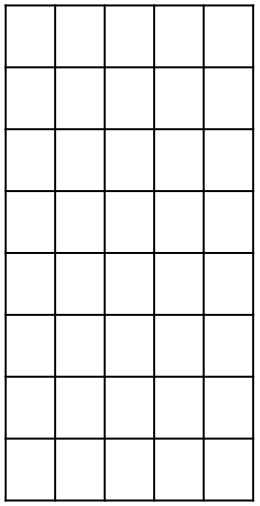Design a fancy curved rooftop design using straight beams:

 In:=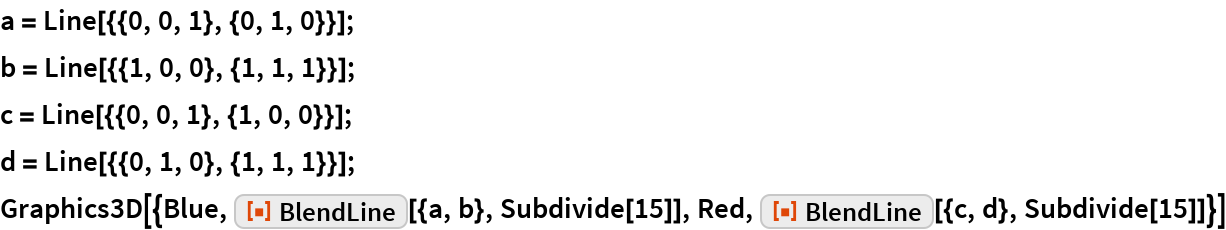Out=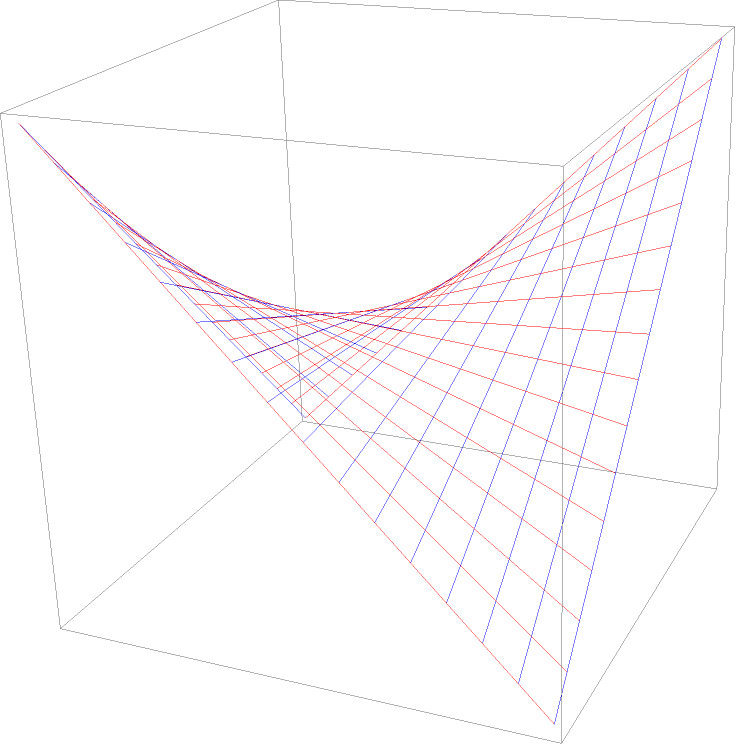### Properties and Relations

Lines do not need the same number of data points. The resulting line can have more points than the original ones:

 In:=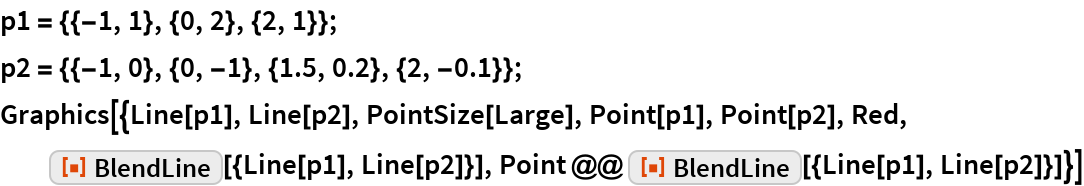Out=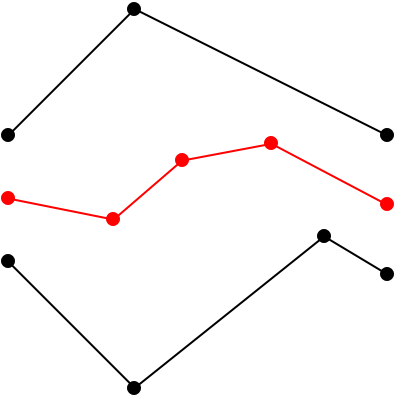Extrapolate beyond the original lines by using numbers outside the range (0,1):

 In:=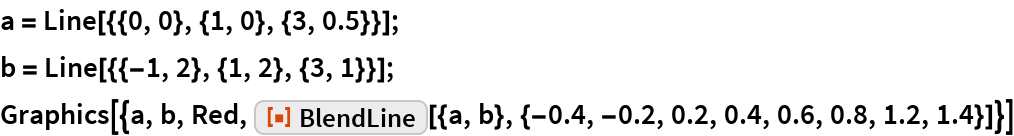Out=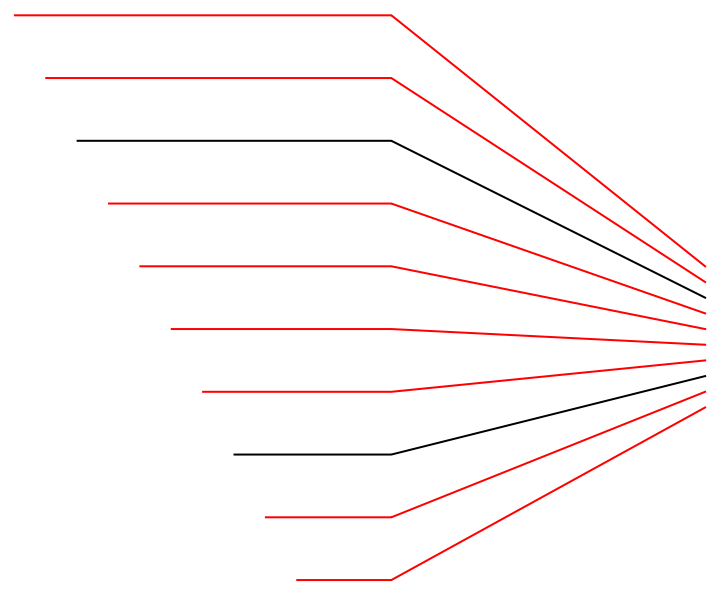### Neat Examples

Interpolate between an octagon and a hexagon:

 In:=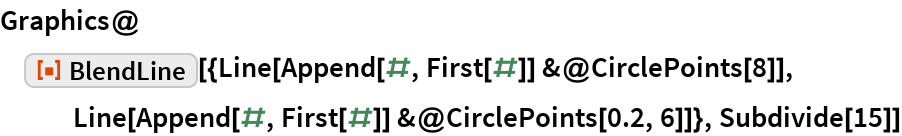Out=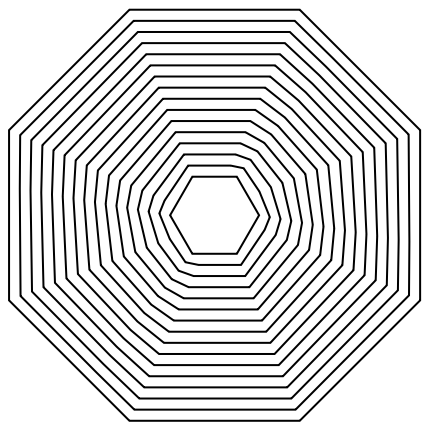Interpolate between a circle and a square:

 In:=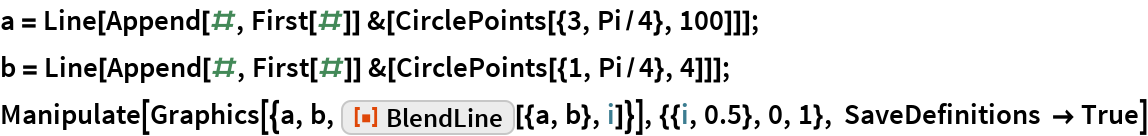Out=